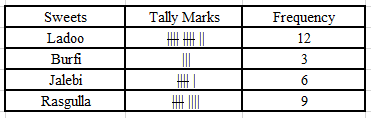## RD Sharma Solutions for Class 6 Chapter 21 Data Handling - I (Presentation of Data) Free Online

Exercise 21.1 page: 21.4
1. Define the following terms:
(i) Observations
(ii) data
(iii) Frequency of an observation
(iv) Frequency distribution
Solution:
(i) The active acquisition of information which is obtained from a primary source is called observation.
(ii) Data is a collection of facts such as values measurements.
(iii) The number of times an observation occurs in the given data, is called the frequency of the observation.
(iv) Frequency table or frequency distribution is a method to present raw data in the form from which one can easily understand the information contained in the raw data.
2. The final marks in mathematics of 30 students are as follows:
53, 61, 48, 60, 78, 68, 55, 100, 67, 90
75, 88, 77, 37, 84, 58, 60, 48, 62, 56
44, 58, 52, 64, 98, 59, 70, 39, 50, 60
(i) Arrange these marks in the ascending order. 30 to 39 one group, 40 to 49 second group, etc.
(ii) What is the highest score?
(iii) What is the lowest score?
(iv) What is the range?
(v) If 40 is the pass mark how many have failed?
(vi) How many have scored 75 or more?
(vii) Which observations between 50 and 60 have not actually appeared?
(viii) How many have scored less than 50?
Solution:
(i) Frequency distribution table of the marks obtained in Mathematics by students: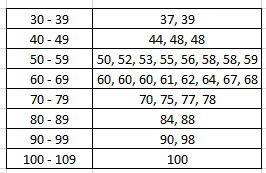(ii) The highest score is 100 from the given data.
(iii) The lowest score is 37 from the above data.
(iv) We know that
Range = Highest score – Lowest score
By substituting the values
Range = 100 – 37 = 63
(v) If 40 is the pass mark, the number of students who scored less than 40 have failed.
Students who scored 37 and 39 have failed.
Hence, 2 students have failed in the exam.
(vi) The number of students who have scored 75 or more are 8 from the data given above.
So the marks obtained by them are 75, 77, 78, 84, 88, 90, 98 and 100.
(vii) Observations 51, 54 and 57 have not actually appeared in the range 50 – 60.
(viii) From the above data we know that 5 students have scored less than 50 i.e. 37, 39, 44, 48 and 48.
3. The weights of new born babies (in kg) in a hospital on a particular day are as follows:
2.3, 2.2, 2.1, 2.7, 2.6, 3.0, 2.5, 2.9, 2.8, 3.1, 2.5, 2.8, 2.7, 2.9, 2.4
(i) Rearrange the weights in descending order.
(ii) Determine the highest weight.
(iii) Determine the lowest weight.
(iv) Determine the range.
(v) How many babies were born on that day?
(vi) How many babies weigh below 2.5 kg?
(vii) How many babies weight more than 2.8 kg?
(viii) How many babies weight 2.8 kg?
Solution:
(i) Rearranging the weights in descending order
3.1, 3.0, 2.9, 2.9, 2.8, 2.8, 2.7, 2.7, 2.6, 2.5, 2.5, 2.4, 2.3, 2.2, 2.1
(ii) The highest weight is 3.1 kg from the given data.
(iii) The lowest weight is 2.1 kg from the given data.
(iv) We know that
Range = Highest weight – Lowest weight
By substituting the values
Range = 3.1 – 2.1 = 1.0 kg
(v) The number of babies born on that day can be found by counting the number of observations.
Hence, 15 babies were born on that day.
(vi) The babies which weigh below 2.5 kg are 2.1, 2.2, 2.3 and 2.4 kg.
Hence, 4 babies weigh below 2.5 kg.
(vii) The babies which weigh more than 2.8 kg are 2.9, 2.9, 3.0 and 3.1 kg.
Hence, 4 babies weigh more than 2.8 kg.
(viii) From the given data we know that 2 babies weigh 2.8 kg.
4. Following data gives the number of children in 40 families:
1, 2, 6, 5, 1, 5, 1, 3, 2, 6, 2, 3, 4, 2, 0, 0, 4, 4, 3, 2
2, 0, 0, 1, 2, 2, 4, 3, 2, 1, 0, 5, 1, 2, 4, 3, 4, 1, 6, 2
Represent it in the form of a frequency distribution.
Solution:
Frequency distribution table for the given data: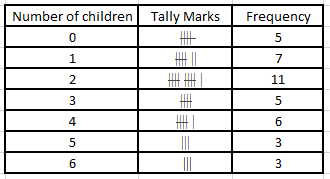5. Prepare a frequency table of the following scores obtained by 50 students in a test:
42 51 21 42 37 37 42 49 38 52
7 33 17 44 39 7 14 27 39 42
42 62 37 39 67 51 53 53 59 41
29 38 27 31 54 19 53 51 22 61
42 39 59 47 33 34 16 37 57 43
Solution:
Frequency distribution of the scores obtained by 50 students in a test is given below: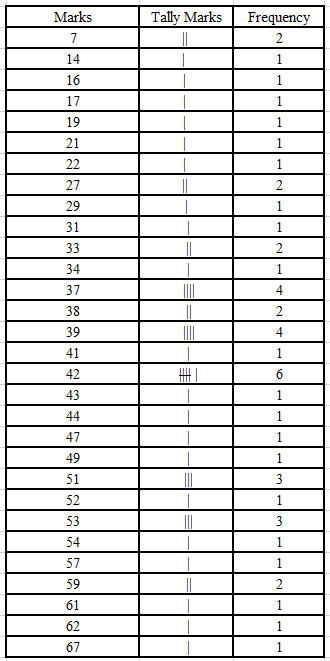6. A die was thrown 25 times and following scores were obtained:
1 5 2 4 3
6 1 4 2 5
1 6 2 6 3
5 4 1 3 2
3 6 1 5 2
Prepare a frequency table of the scores.
Solution:
Frequency table of the given scores: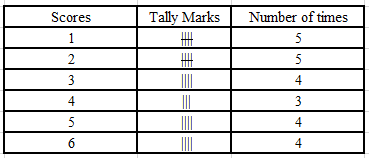7. In a study of number of accidents per day, the observations for 30 days were obtained as follows:
6 3 5 6 4 3 2 5 4 2
4 2 1 2 2 0 5 4 6 1
6 0 5 3 6 1 5 5 2 6
Prepare a frequency distribution table.
Solution:
Frequency distribution table of the number of accidents per day is given: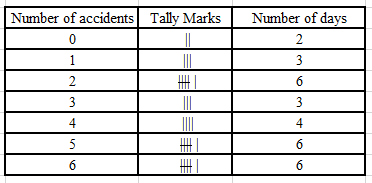8. Prepare a frequency table of the following ages (in years) of 30 students of class VIII in your school:
13, 14, 13, 12, 14, 13, 14, 15, 13, 14, 13, 14, 16, 12, 14
13, 14, 15, 16, 13, 14, 13, 12, 17, 13, 12, 13, 13, 13, 14.
Solution:
Frequency table of the following ages of 30 students is given below: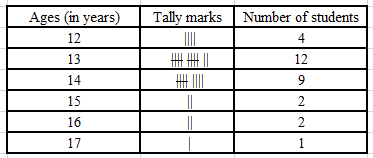9. Following figures relate the weekly wages (in Rs.) of 15 workers in a factory:
300, 250, 200, 250, 200, 150, 350, 200, 250, 200, 150, 300, 150, 200, 250
Prepare a frequency table.
(i) What is the range in wages (in Rs)?
(ii) How many Workers are getting Rs 350?
(iii) How many workers are getting the minimum wages?
Solution:
Frequency table of weekly wages of 15 workers is given below: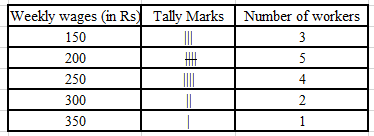(i) From the table we know that Rs 150 is the minimum wages and Rs 350 is the maximum wages given to workers
Range = Maximum wage – Minimum wage
By substituting the values
Range = 350 – 150 = Rs 200
(ii) From the frequency table we know that 1 worker is getting Rs 350.
(iii) We know that Rs 150 is the minimum wage
So the 3 workers getting minimum wage.
10. Construct a frequency distribution table for the following marks obtained by 25 students in a history test in class VI of a school:
9, 17, 12, 20, 9, 18, 25, 17, 19, 9, 12, 9, 12, 18, 17, 19, 20, 25, 9, 12, 17, 19, 19, 20, 9
(i) What is the range of marks?
(ii) What is the highest mark?
(iii) Which mark is occurring more frequently?
Solution:
Frequency table of marks obtained by 25 students is given below: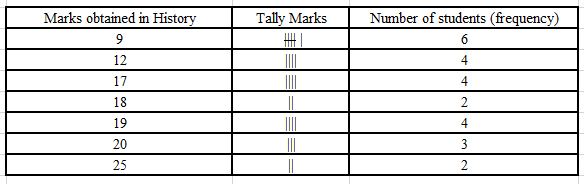(i) From the table we know that 25 is the highest marks and 9 is the lowest marks
Range = Highest marks – Lowest marks
By substituting the values
Range = 25 – 9 = 16
(ii) The highest mark from the frequency table is 25.
(iii) The marks occurring frequently can be found by counting the number of observations.
Hence, 9 occurs more frequently.
11. In a mathematics test following marks were obtained by 40 students of class VI. Arrange these marks in a table using, tally marks.
8 1 3 7 6 5 5 4 4 2
4 9 5 3 7 1 6 5 2 7
7 3 8 4 2 8 9 5 8 6
7 4 5 6 9 6 4 4 6 6
(i) Find how many students obtained marks equal to or more than 7?
(ii) How many students obtained marks below 4?
Solution:
Frequency table of the marks obtained in Mathematics is given below: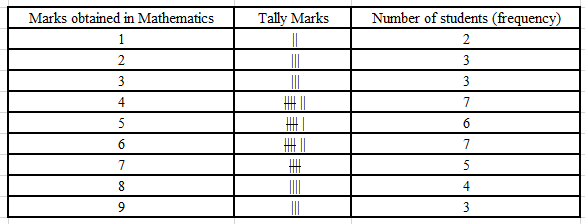(i) No. of students who got marks equal to or more than 7 = Frequency of 7 + Frequency of 8 + Frequency of 9
By substituting the values
No. of students who got marks equal to or more than 7 = 5 + 4 + 3 = 12
(ii) No. of students who got marks below 4 = Frequency of 1 + Frequency of 2 + Frequency of 3
By substituting the values
No. of students who got marks below 4 = 2 + 3 + 3 = 8